# Speed Test Results

## Experiment

The speed test compares the libstdc++ STL red-black tree with the implemented B+ tree with many different parameters. To keep focus on the algorithms and reduce data copying the multiset specializations were chosen. Two set of test procedures are used: the first only inserts n random integers into the tree. The second test first inserts n random integers, then performs n lookups for those integers and finally erases all n integers.

These two test sequences are preformed for n from 125 to 4,096,000 where n is doubled after each test run. For each n the test cycles are run until in total 8,192,000 items were inserted. This way the measured speed for small n is averaged over up to 65,536 sample runs.

Lastly it is purpose of the test to determine a good node size for the B+ tree. Therefore the test runs are performed on different slot sizes; both inner and leaf nodes hold the same number of items. The number of slots tested ranges from 4 to 256 slots and therefore yields node sizes from about 50 to 2,048 bytes. This requires that the B+ tree template is instantiated for each of the probed node sizes!

Attention:
Compilation of the speed test with -O3 can take very long and required much RAM.
The results are be displayed below using gnuplot. All tests were run on a Pentium4 3.2 GHz with 1 GB RAM. A high-resolution PDF plot of the following images can be found in the package at speedtest/speedtest.pdf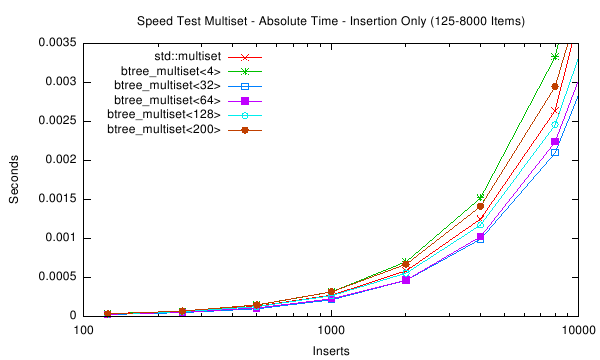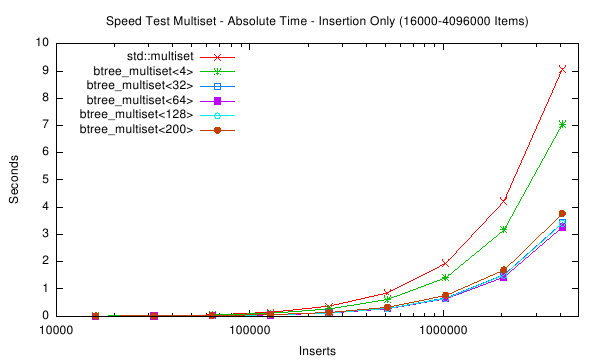The first two plots above show the absolute time measured for inserting n items into six different tree variants. For small n (the first plot) the speed of red-black tree and B+ tree are very similar. For large n the red-black tree slows down, and for n > 1,024,000 items the red-black tree requires almost twice as much time as a B+ tree with 32 slots.

The next plot shows the insertion time per item, which is calculated by dividing the absolute time by the number of inserted items. Notice that insertion time is now in microseconds. The plot shows that the red-black tree reaches some limitation at about n = 16,000 items. Beyond this item count the B+ tree (with 32 slots) performs much better than the STL multiset.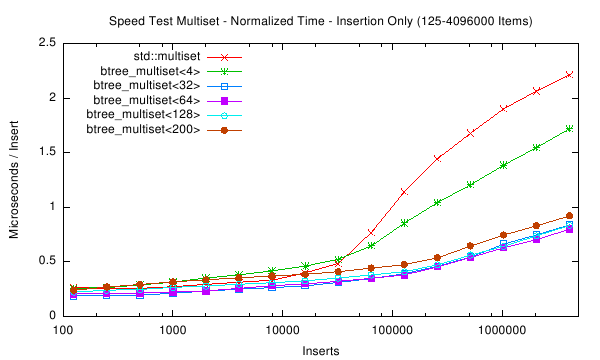The last plots goal is to find the best node size for the B+ tree. It displays the total measured time of the insertion test depending on the number of slots in inner and leaf nodes. The first data point on the left is the running time of the red-black tree. Only runs with more than 1 million inserted items are plotted. One can see that the minimum is around 65 slots for each of the curves. However to reduce unused memory in the nodes the most practical slot size is around 35. This amounts to total node sizes of about 280 bytes. Thus in the implementation a target size of 256 bytes was chosen.

The following four plots show the same aspects as above, except that not only insertion time was measured. Instead a whole insert/find/delete cycle was performed and measured. The results are in general accordance to those of only insertion.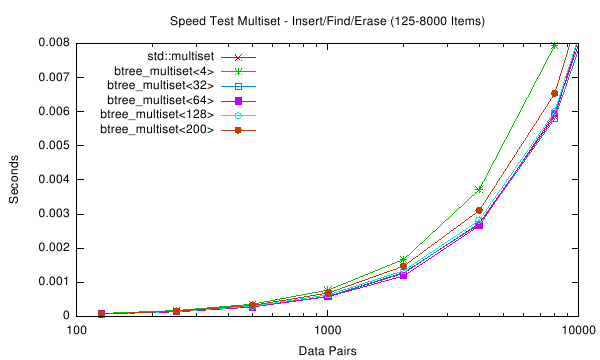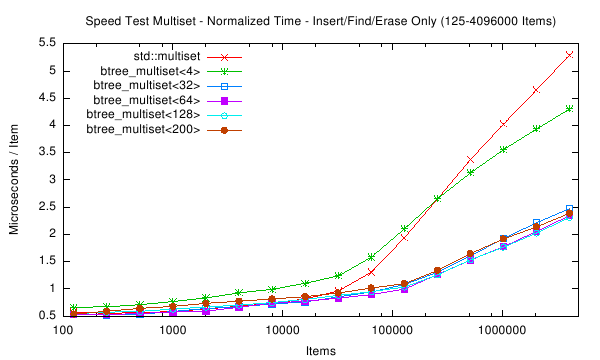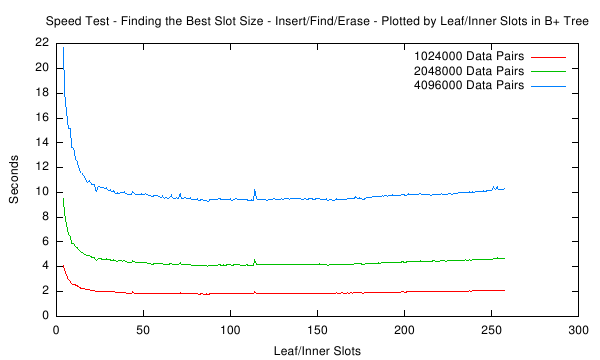Generated on Fri Apr 27 14:49:56 2007 for STX B+ Tree Template Classes by1.5.2